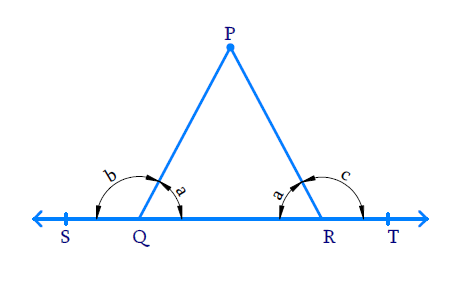# Ex.6.1 Q3 Lines and Angles Solution - NCERT Maths Class 9

Go back to  'Ex.6.1'

## Question

In the given figure, $$\angle PQR = \angle PRQ$$ then prove that $$\angle PQS = \angle PRT.$$Video Solution
Lines And Angles
Ex 6.1 | Question 3

## Text Solution

What is known?

$$\angle PQR = \angle PRQ$$

What is unknown?

To prove $$\angle PQS = \angle PRT$$

Reasoning:

If a ray stands on a line, then the sum of adjacent angles formed is $$180^ {\circ}.$$

steps:

Let $$\angle PQR= \angle PRQ = a$$

Let $$\angle PQS = b$$ and $$\angle PRT = c$$

Line $$ST$$ and $$PQ$$ intersect at point $$Q$$, then the sum of adjacent angles $$\angle PQS$$ and $$\angle PQR$$ is $$180^ {\circ}.$$

\begin{align} \angle PQS + \angle PQR & = 180 ^ { \circ } \\ b + a & = 180 ^ { \circ } \\ b & = 180 ^ { \circ } - a\ldots . ( 1 ) \end{align}

Line $$ST$$ and $$PR$$ intersect at point $$R$$, then the sum of adjacent angles $$\angle PRQ$$ and $$\angle PRT$$ is $$180^ {\circ}.$$

\begin{align} \angle PRQ + \angle PRT & = 180 ^ { \circ } \\ a + c & = 180 ^ { \circ } \\ c & = 180 ^ { \circ } - a\ldots . ( 2 ) \end{align}

From equations ($$1$$) and ($$2$$), it is clear that $$b = c.$$

Hence $$\angle PQS = \angle PRT$$ is proved.

Video Solution
Lines And Angles
Ex 6.1 | Question 3

Learn from the best math teachers and top your exams

• Live one on one classroom and doubt clearing
• Practice worksheets in and after class for conceptual clarity
• Personalized curriculum to keep up with school